Time
What's the hour
Count the minutes
Show the
time
How long until
100
The big hand.What is the minute hand
100What is 5 o'clock
100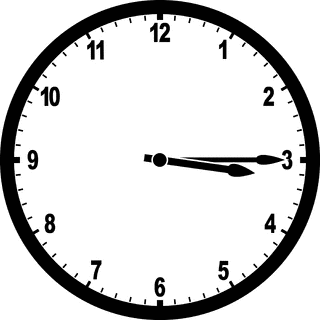What is 15
100
3:50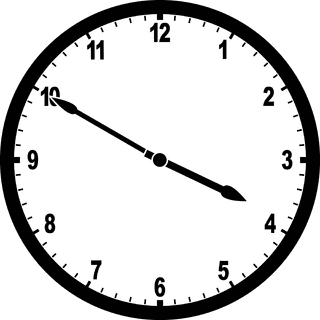1005:30 /
What is 5 minutes
200
The small hand.
What is the hour hand
200What is 3 o'clock
200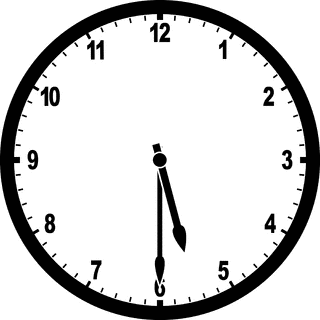What is 30
200
1:30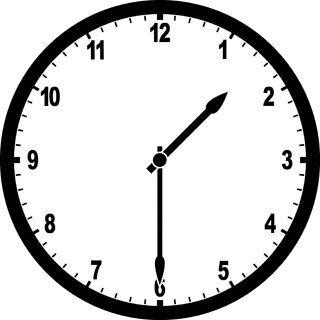2004:00 /
What is 10 minutes
300What is 1:30
300What is 1 o'clock
300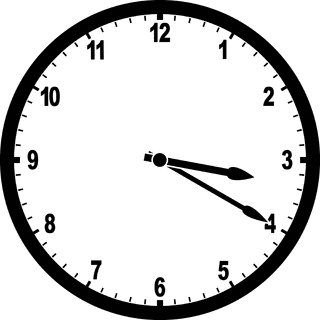What is 20
300
2:45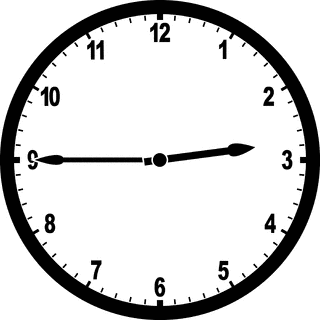3003:00 /
What is 15 minutes
400What is 2:45
400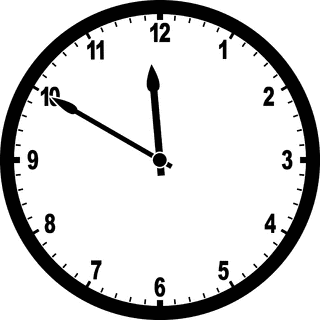What is 11 o'clock
400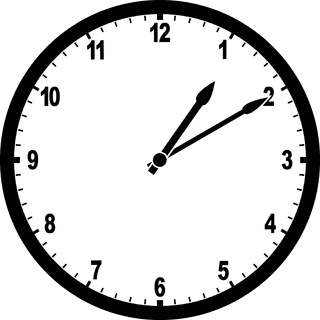What is 10
400
9:504001:45 /
What is 20 minutes
500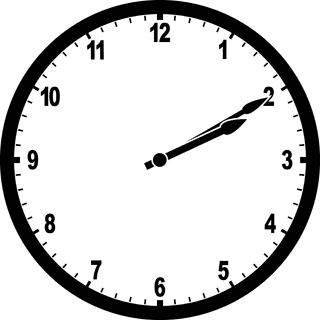What is 2:10
500What is 9 o'clock
500
What is 55
500
3:155002:10 /
What is 1 hour
Click to zoom